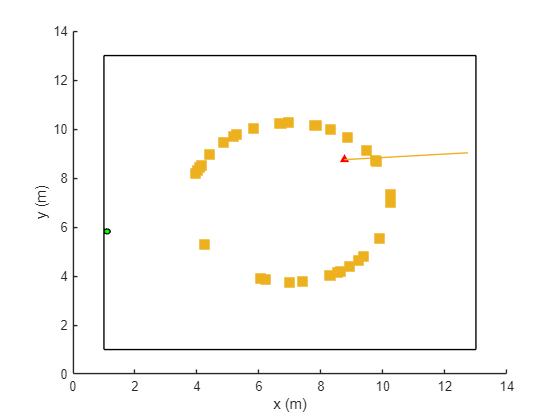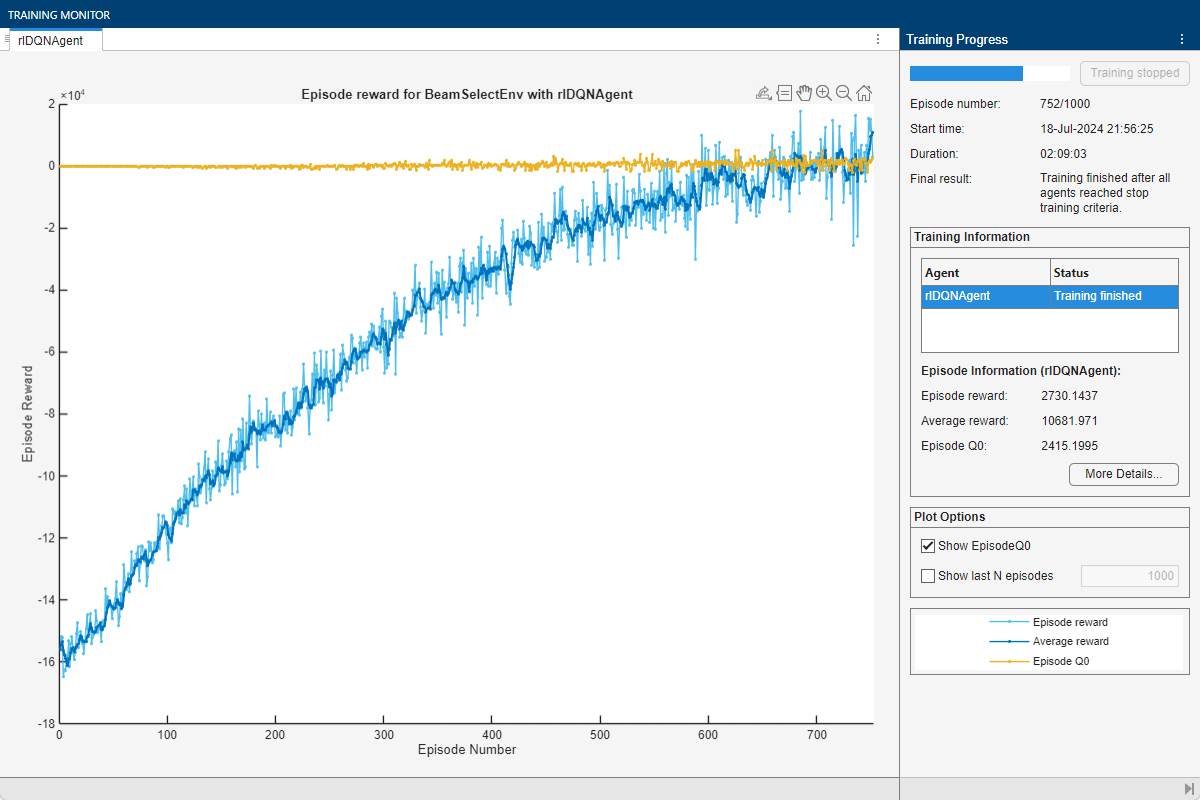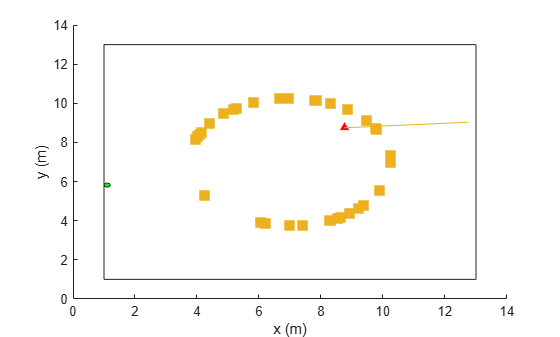# Train DQN Agent for Beam Selection

This example shows how to train a deep Q-network (DQN) reinforcement learning agent to accomplish the beam selection task in a 5G New Radio (NR) communications system. Instead of an exhaustive beam search over all the beam pairs, the trained agent increases beam selection accuracy by selecting the beam with highest signal strength while reducing the beam transition cost. Considering an access network node (gNB) with four beams, simulation results in this example show the trained agent selects beams with greater than 90% maximum possible signal strengths while reducing the beam transition cost.

### Introduction

To enable millimeter wave (mmWave) communications, beam management techniques must be used due to the high pathloss and blockage experienced at high frequencies. Beam management is a set of Layer 1 (physical layer) and Layer 2 (medium access control) procedures to establish and retain an optimal beam pair (transmit beam and a corresponding receive beam) for good connectivity . For examples of NR beam management procedures, see NR SSB Beam Sweeping (5G Toolbox) and NR Downlink Transmit-End Beam Refinement Using CSI-RS (5G Toolbox).

This example considers beam selection procedures when a connection is established between the user equipment (UE) and gNB. In 5G NR, the beam selection procedure for initial access consists of beam sweeping, which requires exhaustive searches over all the beams on the transmitter and the receiver sides, and then selection of the beam pair offering the strongest reference signal received power (RSRP). Since mmWave communications require many antenna elements, implying many beams, an exhaustive search over all beams becomes computationally expensive and increases the initial access time.

To avoid repeatedly performing an exhaustive search and to reduce the communication overhead, this example uses a reinforcement learning (RL) agent to perform beam selection using the GPS coordinates of the receiver and the current beam angle while the UE moves around the track.

In this figure, the square represents the track that the UE (green circle) moves around, the red triangle represents the location of the base station (gNB), the yellow squares represent the channel scatterers, and the blue line represents the selected beam.For more information on DQN reinforcement learning agents, see Deep Q-Network (DQN) Agents (Reinforcement Learning Toolbox).

### Define Environment

To train a reinforcement learning agent, you must define the environment with which it will interact. The reinforcement learning agent selects actions given observations. The goal of the reinforcement learning algorithm is to find optimal actions that maximize the expected cumulative long-term reward received from the environment during the task. For more information about reinforcement learning agents, see Reinforcement Learning Agents (Reinforcement Learning Toolbox).

For the beam selection environment:

• The observations are represented by UE position information and the current beam selection.

• The actions are a selected beam out of four total beam angles from the gNB.

• The reward ${\mathit{r}}_{\mathit{t}}$ at time step $\mathit{t}$ is given by:

`$\begin{array}{l}{\mathit{r}}_{\mathit{t}}={\mathit{r}}_{\mathrm{rsrp}}+{\mathit{r}}_{\theta }\\ {\mathit{r}}_{\mathrm{rsrp}}=0.9×\text{\hspace{0.17em}}\mathrm{rsrp}\\ {\mathit{r}}_{\theta }=-0.1×\text{\hspace{0.17em}}\mathrm{abs}\left({\theta }_{\mathit{t}}-{\theta }_{\mathit{t}-1}\right).\end{array}$`

${\mathit{r}}_{\mathrm{rsrp}}$ is a reward for the signal strength measured from the UE (rsrp) and ${\mathit{r}}_{\theta }$ is a penalty for control effort. $\theta$ is the beam angle in degrees.

The environment is created from the RSRP data generated from the Neural Network for Beam Selection (5G Toolbox) example. In the prerecorded data, receivers are randomly distributed on the perimeter of a 6-meter square and configured with 16 beam pairs (four beams on each end, analog beamformed with one RF chain). Using a MIMO scattering channel, the example considers 200 receiver locations in the training set (`nnBS_TrainingData.mat`) and 100 receiver locations in the test sets (`nnBS_TestData.mat`). The prerecorded data uses 2-D location coordinates.

The `nnBS_TrainingData.mat` file contains a matrix of receiver locations, `locationMatTrain`, and RSRP measurements of 16 beam pairs, `rsrpMatTrain`. Since receiver beam selection does not significantly affect signal strength, you compute the mean RSRP for each base station antenna beam for each UE location. Thus, the action space is four beam angles. The recorded data is reordered to imitate the receiver moving in the clockwise direction around the base station.

To generate new training and test sets, set `useSavedData` to `false`. Be aware that regenerating data can take up to a few hours.

```% Set the random generator seed for reproducibility rng(0) useSavedData = true; if useSavedData % Load data generated from Neural Network for Beam Selection example load nnBS_TrainingData load nnBS_TestData load nnBS_position else % Generate data helperNNBSGenerateData(); %#ok position.posTX = prm.posTx; position.ScatPos = prm.ScatPos; end locationMat = locationMatTrain(1:4:end,:); % Sort location in clockwise order secLen = size(locationMat,1)/4; [~,b1] = sort(locationMat(1:secLen,2)); [~,b2] = sort(locationMat(secLen+1:2*secLen,1)); [~,b3] = sort(locationMat(2*secLen+1:3*secLen,2),"descend"); [~,b4] = sort(locationMat(3*secLen+1:4*secLen,1),"descend"); idx = [b1;secLen+b2;2*secLen+b3;3*secLen+b4]; locationMat = locationMat(idx,:); % Compute average RSRP for each gNB beam and sort in clockwise order avgRsrpMatTrain = rsrpMatTrain/4; % prm.NRepeatSameLoc=4; avgRsrpMatTrain = 100*avgRsrpMatTrain./max(avgRsrpMatTrain, [],"all"); avgRsrpMatTrain = avgRsrpMatTrain(:,:,idx); avgRsrpMatTrain = mean(avgRsrpMatTrain,1); % Angle rotation matrix: update for nBeams>4 txBeamAng = [-78,7,92,177]; rotAngleMat = [ 0 85 170 105 85 0 85 170 170 85 0 85 105 170 85 0]; rotAngleMat = 100*rotAngleMat./max(rotAngleMat,[],"all"); % Create training environment using generated data envTrain = BeamSelectEnv(locationMat,avgRsrpMatTrain,rotAngleMat,position); ```

The environment is defined in the `BeamSelectEnv` supporting class, which is created using the `rlCreateEnvTemplate` class. `BeamSelectEnv.m` is located in this example folder. The reward and penalty functions are defined within and are updated as the agent interacts with the environment.

### Create Agent

A DQN agent approximates the long-term reward for the given observations and actions by using a `rlVectorQValueFunction` (Reinforcement Learning Toolbox) critic. Vector Q-value function approximators have observations as inputs and state-action values as outputs. Each output element represents the expected cumulative long-term reward for taking the corresponding discrete action from the state indicated by the observation inputs.

The example uses the default critic network structures for the given observation and action specification.

```obsInfo = getObservationInfo(envTrain); actInfo = getActionInfo(envTrain); agent = rlDQNAgent(obsInfo,actInfo);```

View the critic neural network.

```criticNetwork = getModel(getCritic(agent)); analyzeNetwork(criticNetwork)```

To foster expoloration, the DQN agent in this example optimizes with a learning rate of 1e-3 and an epsilon decay factor of 1e-4. For a full list of DQN hyperparameters and their descriptions, see `rlDQNAgentOptions` (Reinforcement Learning Toolbox).

Specify the agent hyperparameters for training.

```agent.AgentOptions.CriticOptimizerOptions.LearnRate = 1e-3; agent.AgentOptions.EpsilonGreedyExploration.EpsilonDecay = 1e-4;```

### Train Agent

To train the agent, first specify the training options using `rlTrainingOptions` (Reinforcement Learning Toolbox). For this example, run each training session for at most 500 episodes, with each episode lasting at most 200 time steps, corresponding to one full loop of the track.

```trainOpts = rlTrainingOptions(... MaxEpisodes=500, ... MaxStepsPerEpisode=200, ... % training data size = 200 StopTrainingCriteria="AverageSteps", ... StopTrainingValue=500, ... Plots="training-progress"); ```

Train the agent using the `train` (Reinforcement Learning Toolbox) function. Training this agent is a computationally intensive process that takes several minutes to complete. To save time while running this example, load a pretrained agent by setting `doTraining` to `false`. To train the agent yourself, set `doTraining` to `true`.

```doTraining = false; if doTraining trainingStats = train(agent,envTrain,trainOpts); %#ok else load("nnBS_RLAgent.mat") end```

This figure shows the progression of the training. You can expect different results due to randomness inherent to the training process.### Simulate Trained Agent

To validate the trained agent, run the simulation on the test environment with UE locations that the agent has not seen in the training process.

```locationMat = locationMatTest(1:4:end,:); % Sort location in clockwise order secLen = size(locationMat,1)/4; [~,b1] = sort(locationMat(1:secLen,2)); [~,b2] = sort(locationMat(secLen+1:2*secLen,1)); [~,b3] = sort(locationMat(2*secLen+1:3*secLen,2),"descend"); [~,b4] = sort(locationMat(3*secLen+1:4*secLen,1),"descend"); idx = [b1;secLen+b2;2*secLen+b3;3*secLen+b4]; locationMat = locationMat(idx,:); % Compute Average RSRP avgRsrpMatTest = rsrpMatTest/4; % 4 = prm.NRepeatSameLoc; avgRsrpMatTest = 100*avgRsrpMatTest./max(avgRsrpMatTest, [],"all"); avgRsrpMatTest = avgRsrpMatTest(:,:,idx); avgRsrpMatTest = mean(avgRsrpMatTest,1); % Create test environment envTest = BeamSelectEnv(locationMat,avgRsrpMatTest,rotAngleMat,position);```

Simulate the environment with the trained agent. For more information on agent simulation, see `rlSimulationOptions` and `sim`.

```plot(envTest) sim(envTest,agent,rlSimulationOptions("MaxSteps",100))``````maxPosibleRsrp = sum(max(squeeze(avgRsrpMatTest))); rsrpSim = envTest.EpisodeRsrp; disp("Agent RSRP/Maximum RSRP = " + rsrpSim/maxPosibleRsrp*100 +"%")```
```Agent RSRP/Maximum RSRP = 94.9399% ```

### References

 3GPP TR 38.802. "Study on New Radio Access Technology Physical Layer Aspects." 3rd Generation Partnership Project; Technical Specification Group Radio Access Network.

 Sutton, Richard S., and Andrew G. Barto. Reinforcement Learning: An Introduction. Second edition. Cambridge, MA: MIT Press, 2020.